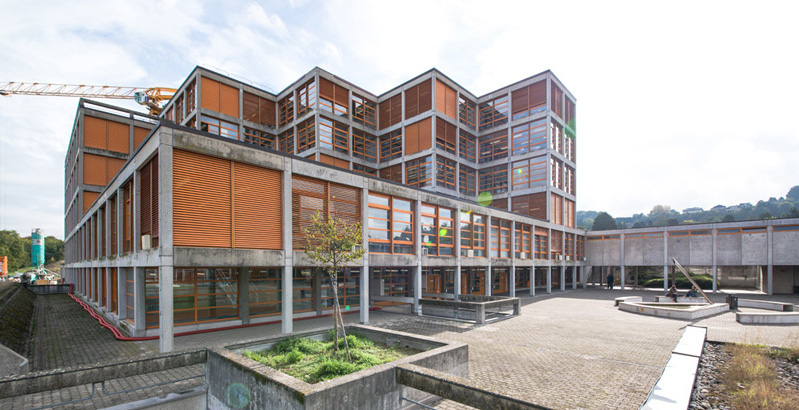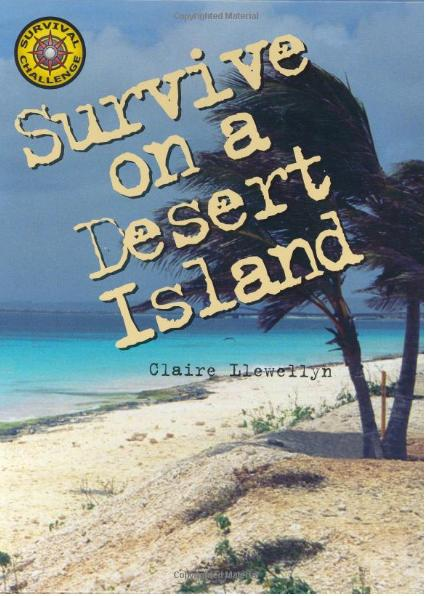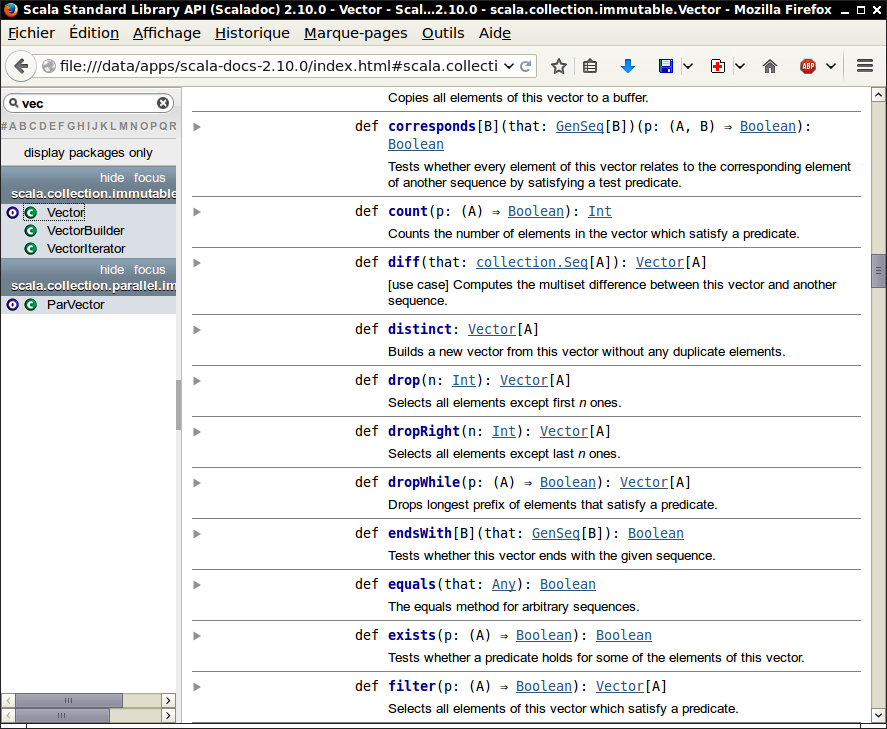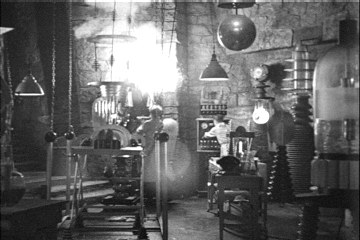# Programmation appliquée en ScalaCopyright © Cay S. Horstmann 2015# Conditional Expressions

• `if (booleanExpression) expression1 else expression2`
• if/else is an expression, not a statement. Can be used in other expressions:
`val y = (if (x >= 0) 2 else 0) - 1`
• Like `? :` in C++
• Type is the most specific supertype common to expression1, expression2
```val z = if (condition) 3 else "Hello"
// type is Any```
• Omitting the `else` yields a value of type `Unit` if `condition` is `false` (like `void` in C++)

# Vectors

• Immutable array
• Random access `v(i)`
• `Vector.fill(n)(e)` yields a new vector with `n` copies of `e`
`val v = Vector.fill(10)("Bonjour")`
• `v.updated(i, e)` yields a new vector with `e` at position `i`
`val v2 = v.updated(9, "Adieu")`
• More common, bulk transformation with `map`
`v2.map(s => s.toLowerCase)`
• Can also pass expression yielding a value to `fill`
`Vector.fill(10)(math.random)`
• `Vector.iterate(s, n)(f)` computes the vector with elements `s`, `f(s)`, `f(f(s))`, ...
`Vector.iterate("Bonjour", 8)(s => s.drop(1))`

# What is the Value of...

`"Fred".toVector.map(c => 'a' <= c && c <= 'z')`
1. `Vector('F', 'r', 'e', 'd')`
2. `Vector('r', 'e', 'd')`
3. `Vector(false, true, true, true)`
4. Something else (other value, syntax error, ...)

# Maps• If I were stranded on a desert island and could only take one data structure with me, it would be the hash table. — Peter van der Linden
• Construct a map
`val scores = Map("Alice" -> 10, "Bob" -> 3, "Cindy" -> 8)`
• Access element
```val alicesScore = scores("Alice")
// They don't call it a map for nothing
val fredsScore = scores.getOrElse("Fred", 0)```
• Update map (i.e. get new map with updates)
```val newScores = scores + ("Bob" -> 10, "Fred" -> 7)
val newerScores = newScores - "Alice" // Remove key and value```

# Tuples

• Heterogeneous sequence of elements
`val myFirstTuple = (1, 3.14, "Fred")`
• Tuple type `(Int, Double, String)`
• Access components with methods `_1` (!), `_2`, `_3`
`val second = myFirstTuple._2`
• Or with destructuring
`val (first, second, _) = myFirstTuple`
• `->` operator makes pairs. `"Alice" -> 10` is the same as `("Alice", 10)`
• A map is a collection of pairs.
```scores.map(p => (p._2, p._1))
scores.map(p => { val (x, y) = p; (y, x) })
// Can't destructure parameter directly
```

# What is `result`...

...where

```val words = "Mary had a little lamb".split(" ").toVector
val result = words.map(w => w -> w.length).toMap```
1. A `Vector[(String, Int)]`
2. A `Vector[String => Int]`
3. A `Map[String, Int]`
4. Something else (other type, syntax error, ...)• At http://www.scala-lang.org/api/2.11.4/
• Tip: Download the docs from the bottom of this site and install on your laptop
• Use the search filter in the top left corner
• Whoa! So many methods!!! Just skip the ones that don't make sense
• C = class (instance methods), O = object (like Java/C++ “static” methods)
• Operators are at the top of each page. Exception: `apply` is the `()` operator, `unary_-` is what you think it is.
• `GenSeq`? `TraversableLike`? `FilterMonadic`??? Just think “some stuff for collections” and move on.
• `implicit` means there is an automatic conversion. Ignore.
• Also look into `RichInt`, `RichDouble`, `StringOps` for numbers and strings
• Math functions are in the package `scala.math`

# Lab• Format of classes: approx. 20 minutes lecture, 40 minutes lab, 15 minute wrap-up
• You work with a buddy
• One of you (the coder) writes the code, the other (the scribe) types up answers
• When you get stuck, ask your buddy first!
• Switch coder/scribe roles each lab
• The coder submits worksheet to Fatemeh.Borran@heig-vd.ch. Include the scribe's name in the worksheet!
• The scribe submits answers to Fatemeh.Borran@heig-vd.ch. Include the coder's name in the report!

# Part 1: If Expressions

1. Make a worksheet `Day2`. Paste in
`if (math.random > 0.5) "Bonjour" else "Adieu"`
and save. What do you get?
2. Paste it in a couple more times. What do you get?
3. Make a vector with ten results of that call. (Not by pasting it 10 times, of course.)
4. Given a sequence of integers, produce a sequence of tuples `(n, 0)` if `n` is positive and `(0, -n)` if `n` is negative. Test with `-10.to(10)`.
5. Write a function that yields 1 if `x > 0` , 0 if `x` equals 0, and `-1` if `x < 0`. Complete `val sign = ...` and then call `-10.to(10).map(sign)`.
6. Do not use any constructs that weren't covered! For example, don't use `def` in the preceding assignment.

# Part 2: Strings are Sequences Too

1. What is `"Fred"(2)`? Why?
2. How can you turn it into `"Free"`? That is, what call yields a new string with characters `'F' 'r' 'e' 'e'`? (Hint: Don't use `substring`, but look at the title of the slide.)
3. How can you reverse a string? Tip: Don't hesitate to experiment in the worksheet. That's what it's there for. Type `"Bonjour".rev` and hit Ctrl Space. What happens?
4. Does that method work for other sequences too? Experiment with `1.to(10)`. What happens?
5. Does `distinct` work with strings? What does it do? Give an example.
6. What about `map`? Give an example.
7. How do you remove the last character of a string? Hint: You saw earlier how to drop the first character. Look up the Scaladoc of `StringOps`.

# Part 3: Fun with Vectors

1. What is
`val words = io.Source.fromFile("/usr/share/dict/words").getLines().toVector`
(If you get an exception that you don't have `/usr/share/dict/words`, then first save the contents of this link somewhere and change the location to something like `"C:\\Users\\Yourname\\Downloads\\words.txt"`.)
2. How many words are in it? (Try out the obvious, or look into Scaladoc.)
3. What is the median entry? Or the two median ones if the length was even.
4. What are the distinct word lengths? (Go back to lab 1 if you need a hint.)
5. Which ones have maximum length? (Hint: Check out the Scaladoc for `filter`.)
6. More filtering. Find all palindromes (i.e. strings that equal their reverse). Hint: In Scala, you compare strings with `==`.

# Part 4: Misery with Tuples

1. What is `1 -> 2 -> 3`? What is the type of the result?
2. Write a function `flatten` that takes such a thing and turns it into an `(Int, Int, Int)`. Use `_1`, `_2`
3. Repeat with destructuring.
4. What is the result of the following statement, and why?
`val x,y,z = (1,2,3)`

# Part 5: If I Had Just One Data Structure...

1. And if I could just take one method, it would be `groupBy`. What is `words.groupBy(w => w.length)`?
2. Make a map so that `myMap('a')` yields all words that start with the letter a and so on.
3. How many words start with a given letter? Hint: `myMap.map(...)`
4. Extra credit: Turn this into a list of tuples that is sorted by frequency. Hint: `sorted` doesn't work because Scala doesn't know how to sort the tuples. There are two other `sort` methods. One of them wants a function that maps into something that Scala does know how to sort—the easy choice for solving this exercise.
5. Extra credit: Now do it with the other method (and some tuple misery).

# Homework

Do this as individual work, not with your partner

When all done, email the signed zip files to Fatemeh.Borran@heig-vd.ch